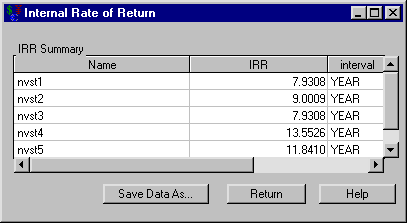# Analyses

### Computing an Internal Rate of Return

You are choosing between five investments. A portfolio containing these investments is available at SASHELP.INVSAMP.NVST. Which investments are acceptable if you expect a MARR of 9%?

Open the portfolio SASHELP.INVSAMP.NVST and compare the investments. Note that Internal Rate of Return computations assume regular periodicity of the cashflow. To compute the internal rates of return, follow these steps:

1. Select all five investments.

2. Select AnalyzeInternal Rate of Return.

Figure 58.4: Computing an Internal Rate of ReturnThe results displayed in Figure 58.4 indicate that the internal rates of return for investments 2, 4, and 5 are greater than 9%. Hence, each of these is acceptable.